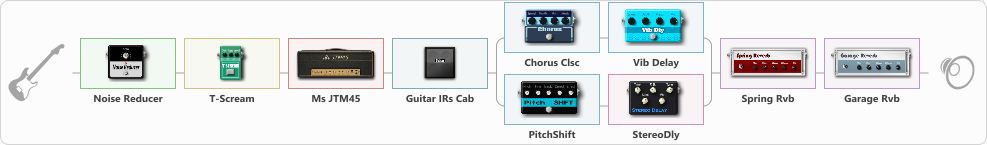# Marshall Jtm truss

Discussion in 'ToneLib-GFX presets' started by truss van halen, Nov 24, 2020.

1. ### truss van halenWell-Known Member

Marshall Jtm truss

Preset name: Marshall JtM truss

Effects chain:Effect: "Noise Reducer" (Dynamics / Filter), active - "yes"
{
"Sens" = 100
"Mode" = Hard
}

Effect: "T-Scream" (Overdrive / Distortion), active - "yes"
{
"Drive" = 24
"Tone" = 53
"Level" = 62
}

Effect: "Ms JTM45" (Amp simulators), active - "yes"
{
"Gain" = 77
"Bass" = 57
"Middle" = 65
"Treble" = 62
"Presence" = 59
"Master" = 100
"Level (dB)" = 0
}

Effect: "Guitar IRs Cab" (Cabinets), active - "yes"
{
"Model" = Engl Pro (4x12")
"Mic Position" = Middle
"Mic Distance" = Near
"Low Cut (Hz)" = 60
"Hi Cut (kHz)" = 20.0
"Mix" = 100
"Level (dB)" = 0
}

Effect: "Splitter" (Dynamics / Filter), active - "yes"
{
"A-Bypass" = Off
"A-Pan" = 0
"A-Level" = 55
"B-Bypass" = Off
"B-Pan" = 0
"B-Level" = 55

'A' branch:
{

Effect: "Chorus Clsc" (Modulation / Sfx), active - "yes"
{
"Speed" = 3.5
"Depth" = 58
"Center" = 1.0
"Mode" = Stereo
}

Effect: "Vib Delay" (Modulation / Sfx), active - "yes"
{
"Speed" = 4.2
"Depth" = 0
"Feedback" = 38
"Time" = 1.6
"Mix" = 100
"Mode" = 1
}
}
'B' branch:
{

Effect: "PitchShift" (Modulation / Sfx), active - "yes"
{
"Pitch" = 7
"Fine" = 8
"Tracking" = 85
"Direct" = 51
"Effect" = 38
}

Effect: "StereoDly" (Delay), active - "yes"
{
"Time" = 756
"Feedback" = 50
"Tone" = 28
"Sens" = 0
"Mix" = 68
}
}
}

Effect: "Spring Rvb" (Reverberation), active - "yes"
{
"Time" = 5.1
"PreDelay" = 0
"LoDamp" = 16
"HiDamp" = 26
"Mix" = 20
}

Effect: "Garage Rvb" (Reverberation), active - "yes"
{
"Time" = 5.2
"PreDelay" = 46
"LoDamp" = 0
"HiDamp" = 30
"Mix" = 43
}

Note: You will need to download and install the ToneLib-GFX software to use the preset.

#### Attached Files:

• ###### Marshall_Jtm_truss.tlgfx
File size:
1 KB
Views:
2,393
Jack Disney likes this.